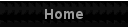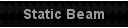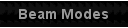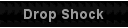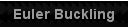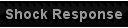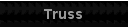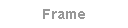The 3D frame calculator determines the frame element loads given the user input loads and boundary conditions at the applicable nodes. The chart shows both the unloaded
and loaded configurations as well as the input loads and global X,Y,Z axes.  The deflected shape can be user scaled and the configuration user rotated about the 3 global axes.

Up to 20 segments can be defined with each having a different elastic modulus, cross-sectional and area moments of inertia. If the frame has more than one segment with the
same elastic modulus, cross-sectional area and area moments of inertia only the first segment values need to be input as the program will assume that the subsequent
segments will have the same value(s).

Discrete boundary conditions of displacements, slopes and concentrated loads can be input.  X, Y and Z are the nodal coordinates in the global coordinate system.  ∆ and θ the
nodal boundary conditions.  P the nodal concentrated loads.  E the elastic modulus, G the shear modulus, I the area moment of Inertia, J the torsional constant and A the cross-
sectional area.
To orient each segment, a 3rd node in the global coordinates is used to locate a point in the local element x-z plane.  The local element x-x axis is from Node i to
Node j.
Note: For the two nodes defining the element the i node number must be < the j node number.

The output includes global nodal deflections and local member axial loads (P), shear loads (V), torsion loads (T) and bending moments (M).
Any consistent set of dimensional units can be used.  Angles are in radians.

The module is dimensional unit neutral. The only requirement is for the units to be consistent with each other.  The only exception is the angle unit for the rotation which is in
radians.  A couple of common groups of units are (inches, pounds, psi, inch-pounds, pounds/inch, inch-pound/radian....) and (mm, Newtons, MPa, mm-N, N/mm, mm-
N/radian....).
 © Copyright 2019 by XL4Sim™All rights reserved.Microsoft® and Microsoft Excel® are registered trademarks of Microsoft Corporation.Terms of Use     Disclaimer     Contact